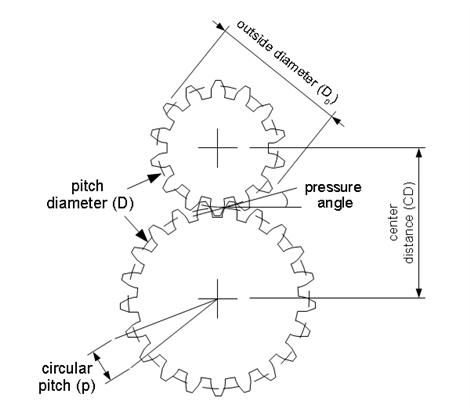# What is the radius of a gear?

I want to find how far a gear from a rack and pinion will rotate from the displacement of the rack and the angle that it will have turned using: s=r*angular displacement.

So what is the radius in this case?

## Answers and Replies

berkeman
Mentor
I want to find how far a gear from a rack and pinion will rotate from the displacement of the rack and the angle that it will have turned using: s=r*angular displacement.

So what is the radius in this case?
Can you post a diagram to make this a bit clearer please? Also, is this for schoolwork?

Can you post a diagram to make this a bit clearer please? Also, is this for schoolwork?

Its a project. I don't know which type of gear I will be using yet, I just want to know what you would use for the radius in that formula in the case of a gear. Would you use the radius of gyration, using the outer and inner radius formula?Nidum
Science Advisor
Gold Member
Gear motion calculations are based on pitch circle diameter - diameter D in your diagram

Gear motion calculations are based on pitch circle diameter - diameter D in your diagram
So I couldn't use the formula s=r*angular displacement to work out the rotation of a rack and pinion gear?

Nidum
Science Advisor
Gold Member
Does that have formulas to work out displacement?

Nidum
Science Advisor
Gold Member
The effective motion geometry of a rack and pinion is that of a plain cylinder of diameter equal to the gear pitch circle diameter rolling without slip against a flat surface .

The effective motion geometry of a rack and pinion is that of a plain cylinder of diameter equal to the gear pitch circle diameter rolling without slip against a flat surface .
So the linear displacement of the rack is equal to the linear displacement of the gear? If so I should be able to use the formula I mentioned?

Nidum
Science Advisor
Gold Member
Linear displacement of rack = ( angle turned by pinion / 360 ) x Pi x pitch circle diameter .

or

Linear displacement of rack = ( angle turned by pinion / 360 ) x Pi x 2 x pitch circle radius .

•Parsifal1
Nidum
Science Advisor
Gold Member
You can also derive formulas based directly on gear module number rather than on pitch circle diameter/radius

Mech_Engineer
Science Advisor
Gold Member
The rack's displacement is equal to the tangential displacement (arc length) of the pinion at the pitch diameter. So if the pitch diameter of the pinion is 20 mm (radius of 10 mm), and you rotate the pinion 90 degrees (π/2 radians), the rack will move about 15.71 mm (calculation here).

•Parsifal1
The rack's displacement is equal to the tangential displacement (arc length) of the pinion at the pitch diameter. So if the pitch diameter of the pinion is 20 mm (radius of 10 mm), and you rotate the pinion 90 degrees (π/2 radians), the rack will move about 15.71 mm (calculation here).

I see, so that was the formula I mentioned then. Problem solved.

Baluncore
Science Advisor
So what is the radius in this case?
You do not need to measure the pitch circle radius. There is an easier way.
You only need to know the pitch of the linear gear rack and the number of teeth on the gear wheel.
That will give you the relationship between linear position and rotation angle.